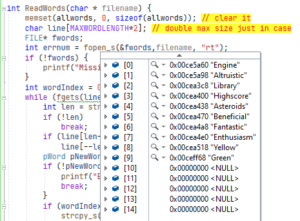### How to read a text file into memory in CAs part of the crossword grid packing utility, the first stage is building the list of words in a structure in RAM. To keep thing simple I’m going to use an array of char * pointers. The array is limited to a maximum 20 words. If any more are read then they will be discarded.

Just to prove that it worked, I wrote this in Visual Studio and ran it in the debugger. You can see elements 0-9 in the screenshot with the source code in the background.

This is the listing of the program. Note, it doesn’t have a function to free up the memory dynamically allocated for each of the words.

You might wonder about these lines (25 and 26).

``````	if (line[len-1] == '\n')
line[--len] = '	if (line[len-1] == '\n')
line[--len] = '\0';';``````

This is needed because when you use fgets to read a line of text it includes a line feed at the end. So the first line in the file Engine is actually 7 characters long with a terminating \n. The malloc on line 27 allocated enough RAM for len + 1 to allow for the terminating 0 at the end of each string. In line 33, if you are on Linux replace strcpy_s with strncpy. Both are safe versions of strcpy. Here’s the full listing.

``````#include <stdio.h>
#include <stdlib.h>
#include <string.h>
#include <time.h>

#define MAXWORDS 20
#define MAXWORDLENGTH 32

typedef char* pWord;
pWord allwords[MAXWORDS];

memset(allwords, 0, sizeof(allwords)); // clear it
char line[MAXWORDLENGTH*2]; // double max size just in case
FILE* fwords;
int errnum = fopen_s(&fwords,filename, "rt");
if (!fwords) {
printf("Missing word file %s", filename);
}
int wordIndex = 0;
while (fgets(line, MAXWORDLENGTH, fwords)) {
int len = strlen(line);
if (!len)
break;
if (line[len-1] == '\n')
line[--len] = '#include <stdio.h>
#include <stdlib.h>
#include <string.h>
#include <time.h>
#define MAXWORDS 20
#define MAXWORDLENGTH 32
typedef char* pWord;
pWord allwords[MAXWORDS];
memset(allwords, 0, sizeof(allwords)); // clear it
char line[MAXWORDLENGTH*2]; // double max size just in case
FILE* fwords;
int errnum = fopen_s(&fwords,filename, "rt");
if (!fwords) {
printf("Missing word file %s", filename);
}
int wordIndex = 0;
while (fgets(line, MAXWORDLENGTH, fwords)) {
int len = strlen(line);
if (!len)
break;
if (line[len-1] == '\n')
line[--len] = '\0';
pWord pNewWord = (pWord)malloc(len + 1);    // line 27
if (!pNewWord) {
printf("Error allocating memory");
break;
}
if (wordIndex < MAXWORDS) { // can add to list
strcpy_s(pNewWord, len+1, line);  // line 33
allwords[wordIndex++] = pNewWord;
}
}
fclose(fwords);
return wordIndex; // = Count of words
}
int main() {
}';
pWord pNewWord = (pWord)malloc(len + 1);    // line 27
if (!pNewWord) {
printf("Error allocating memory");
break;
}
if (wordIndex < MAXWORDS) { // can add to list
strcpy_s(pNewWord, len+1, line);  // line 33
allwords[wordIndex++] = pNewWord;
}
}
fclose(fwords);
return wordIndex; // = Count of words
}

int main() {